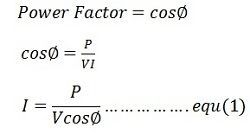# Power Factor

The power factor is defined as the ratio of the active power (P) and volt-amperes. The active power is the real power which is assumed in an AC circuit, whereas volt-amperes is the apparent power which is produced in the circuit when the waves of voltage or current are not in phase.For sinusoidal waveforms, the power factor is the cosine of the angle (phase angle) between voltage and current.Equation (1)  shows that the current is affected by the power factor. Hence, for a given power P by the load, the current I, taken by the load varies inversely as the load power factor cosΦ. Thus, a given load takes more current at a low power factor than it does at a high power factor.

### Disadvantages of low power factor

The undesirable effect of operating a low load at a low power factor is due to the large current required for a low power factor. The important disadvantages of low power factor are

• Higher current is required by the equipment,  due to which the economic cost of the equipment is increased.
• At low power factor, the current is high which gives rise to high copper losses in the system and therefore the efficiency of the system is reduced.
• Higher current produced a large voltage drop in the apparatus. This results in the poor voltage regulation.

Since both the capital and running cost are increased, the operation of the system at low power factor (whether it is lagging or leading) is uneconomical from the supplier’s point of view.

### Causes of Low power factor

The usual reason for the low power factor is because of the inductive load. The current in the inductive load lag behind the voltage. The power factor is therefore lagging. The important inductive loads responsible for the low power factor are the three-phase induction motors  (which operate at a 0.8 lagging power factor), transformer, lamps and welding equipment operate at low lagging power factors. Power factor improvement methods are used for improving the value of power factor in a power system.